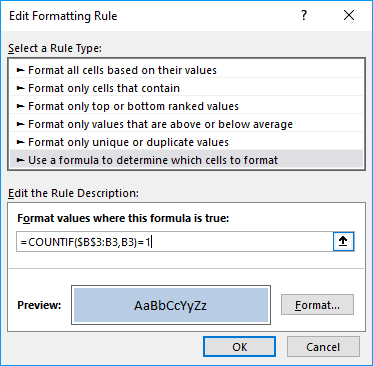Author: Oscar Cronquist Article last updated on January 09, 2019

In this blog post I will demonstrate a conditional formatting formula that will highlight common records in two lists. The image above shows you highlighted records in List 1 that also exists in sheet2.

You can also use a formula to extract shared records or an Excel defined table, if you prefer that. There is also an article written for� Comparing two columns and highlight values in common.

There are more links to related articles in the sidebar.

### Create named ranges

When constructing a conditional formatting formula you can only reference cells on current sheet. But there is a workaround.� The answer is� named ranges.

Here is how to create named ranges for this example:

1. Go to sheet List 2
2. Select cell range A2:A13
3. Click in Name box
4. Type a name (Year)
5. Repeat step 1 to 4 with remaining columns.

### Setup COUNTIFS function

The COUNTIFS function looks for duplicate records in the second list (List 2).

COUNTIFS(criteria_range1,criteria1, criteria_range2,� criteria2...)
Counts the number of cells specified by a given set of conditions or criteria

In this example:
List 1:Column A - List 2: Column A (Year)
List 1:Column B - List 2: Column B (Asset)
List 1:Column C - List 2: Column C (Cost)

COUNTIFS(YEAR, \$A2, ASSET, \$B2, COST, \$C2)

This formula contains absolute and relative cell references. The conditional formatting formula must look for values in matching columns.

\$A2 is a cell reference to values in column A. The column (\$A) is absolute but the row (2) is relative. When the conditional formatting formula executes the next row, only the row number changes, example \$A3. The named ranges do not change.

If the COUNTIFS function finds one matching record it returns 1, if nothing is found 0 (zero) is returned. 0 (zero) is the equivalent to FALSE so the Conditional formatting� will not highlight a cell i FALSE is returned.

### Highlight common records from two lists Excel 2007

How to apply conditional formatting formula:

1. Select cells A2:C11 (Sheet: List 1)
2. Click "Home" tab
3. Click "Conditional Formatting" button
4. Click "New Rule.."
5. Click "Use a formula to determine which cells to format"
6. Type =COUNTIFS(YEAR, \$A2, ASSET, \$B2, COST, \$C2)� in "Format values where this formula is TRUE" window.(The formula shown in the image above is not used in this article)
7. Click "Format.." button
8. Click "Fill" tab
9. Select a color for highlighting cells.
10. Click "Ok"
11. Click "Ok"
12. Click "Ok"

### Highlight common records from two lists Excel 2003

Conditional formatting formula:

=SUMPRODUCT(COUNTIF(\$A2, Year)*COUNTIF(\$B2, Asset)*COUNTIF(\$C2, Cost))